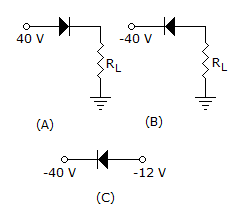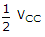# Electronics and Communication Engineering - Analog Electronics

6.

In each of circuits below, indicate which diode is conducting?A. Figure A B. Figure B C. Figure C D. All the three

Explanation:

No answer description available for this question. Let us discuss.

7.

A dc amplifier can operate

 A. at zero frequency B. only at low frequency C. only at high frequencies D. both at low and high frequencies

Explanation:

No answer description available for this question. Let us discuss.

8.

Assertion (A): In a common source amplifier with source terminal at ac ground the voltage gain is about gm rd

Reason (R): A common source amplifier is a source follower circuit

 A. Both A and R are correct and R is correct explanation for A B. Both A and R are correct but R is not correct explanation for A C. A is correct R is wrong D. A is wrong R is correct

Explanation:

No answer description available for this question. Let us discuss.

9.

Thermal runaway will take place if the quiescent point is such that

 A. VCE >B. VCE < VCC C. VCE < 2VCC D. VCE <Explanation:

No answer description available for this question. Let us discuss.

10.

In applications where __________ input resistance is needed, the JFET is preferred to the bipolar transistor.

 A. zero B. very low C. low D. high